International
Tables for
Crystallography
Volume C
Mathematical, physical and chemical tables
Edited by E. Prince

International Tables for Crystallography (2006). Vol. C, ch. 4.2, pp. 192-193

## Section 4.2.1.2. The continuous spectrum

U. W. Arndta

#### 4.2.1.2. The continuous spectrum

| top | pdf |

The shape of the continuous spectrum from a thick target is very simple: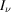, the energy per unit frequency band in the spectrum, is given by the expression derived by Kramers (1923):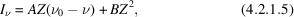where Z is the atomic number of the target and A and B are constants independent of the applied voltage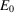. B/A is of the order of 0.0025 so that the term in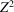can usually be neglected (Fig. 4.2.1.3).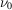is the maximum frequency in the spectrum, i.e. the Duane–Hunt limit at which the entire energy of the bombarding electrons is converted into the quantum energy of the emitted photon, where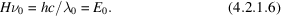Using the latest adjusted values of the fundamental constants (Cohen & Taylor, 1987):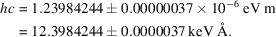Equation (4.2.1.5)can be rewritten in a number of forms. If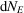is the number of photons of energy E per incident electron,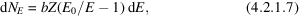where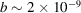photons eV−1  electron−1, and is known as Kramer's constant.Figure 4.2.1.3 | top | pdf |Intensity per unit frequency interval versus frequency in the continuous spectrum from a thick target at different accelerating voltages. From Kuhlenkampff & Schmidt (1943).

From (4.2.1.7), it follows that the total energy in the continuous spectrum per electron is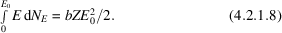Since the energy of the bombarding electron is, the efficiency of production of the continuous radiation is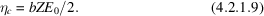Crystallographers are more accustomed to thinking of the spectrum in terms of wavelength. Equation (4.2.1.7)can be transformed into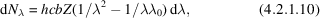which has a maximum at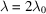. In practice, the emerging spectrum is modified by target absorption, which is greatest for the longer wavelengths and moves the maximum more nearly to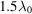.

It is of interest to compare the X-ray flux in a narrow wavelength band selected by an appropriate monochromator with the flux in a characteristic spectral line, in order to examine the practicability of XAFS (X-ray absorption fine-structure spectroscopy) or optimized anomalous-dispersion diffractometry experiments. For these purposes, the maximum permissible wavelength band is about 10−3 Å. From equation (4.2.1.10), we see that, for a tungsten-target X-ray tube operated at 80 kV,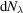is about 1.1 × 10−5 photons with the Kα energy electron−1 steradian−1 (10−3 δλ/λ)−1 for an X-ray wavelength in the neighbourhood of 1.5 Å. By comparison, from equation (4.2.1.2), a copper-target tube operated at 40 kV produces about 5 × 10−4 Kα photons electron−1 steradian−1. In spite of this shortcoming by a factor of about 45, laboratory XAFS experiments are sufficiently common to have merited at least one specialized conference (Stern, 1980; see also Tohji, Udagawa, Kawasak & Masuda, 1983; Sakurai, 1993; Sakurai & Sakurai, 1994).

The use of continuous radiation for diffraction experiments is complicated by the fact that the radiation is polarized. The degree of polarization may be defined as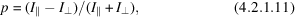where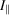and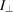are the intensities of radiation with the electric vector parallel and perpendicular to the plane containing the incident electrons and the direction of the emitted photons. For an angle of π/2 between the electrons and the emitted beam, p varies smoothly through the spectrum; it is negative for the softest radiation, approximately zero at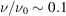and reaches values between +0.7 and +0.9 near the Duane–Hunt limit (Kirkpatrick & Wiedmann, 1945). Since practical use of white radiation is likely to be in the vicinity of, the effect is not a large one.

It should also be noted that the spatial distribution of the white spectrum, even after correction for absorption in the target, is not isotropic. The intensity has a maximum at about 50° to the electron beam and non-zero minima at 0 and 180° to that beam (Stephenson, 1957).

### References

Cohen, E. R. & Taylor, B. N. (1987). The 1986 adjustment of the fundamental physical constants. Rev. Mod. Phys. 89, 1121–1148.Google Scholar
Kirkpatrick, P. & Wiedmann, L. (1945). Theoretical continuous X-ray energy and polarization. Phys. Rev. 67, 321–339.Google Scholar
Kramers, H. A. (1923). On the theory of X-ray absorption and of the continuous X-ray spectrum. Philos. Mag. 46, 836–871.Google Scholar
Kulenkampff, H. & Schmidt, L. (1943). Die Energieverteilung im Spektrum der Röntgen Bremsstrahlung. Ann. Phys. (Leipzig), 43, 494–512.Google Scholar
Sakurai, K. (1993). High-intensity X-ray line-focal spot for laboratory EXAFS measurements. Rev. Sci. Instrum. 64, 267–268.Google Scholar
Sakurai, K. & Sakurai, H. (1994). Comment on high intensity low tube-voltage X-ray source for laboratory EXAFS measurements. Rev. Sci. Instrum. 65, 2417–2418.Google Scholar
Stephenson, S. T. (1957). The continuous X-ray spectrum. Handbuch der Physik. XXX: X-rays, edited by S. Flügge, pp. 337–370. Berlin: Springer.Google Scholar
Stern, E. A. (1980). Editor. Laboratory EXAFS facilities 1980. AIP Conf. Proc. No. 64.Google Scholar
Tohji, K., Udagawa, Y., Kawasaki, T. & Masuda, K. (1983). Laboratory EXAFS spectrometer with a bent-crystal, a solid-state detector, and a fast detection system. Rev. Sci. Instrum. 54, 1482–1487.Google Scholar